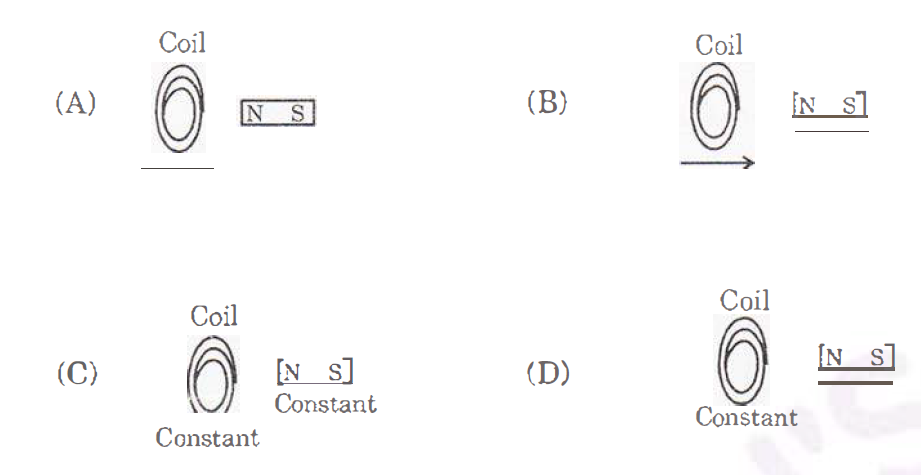# Telangana SSC Board Question Paper for Class 10th General Science Part 1 2019 In PDF

## Telangana 10th Standard General Science Part 1 Exam Question Paper 2019 with Solutions – Free Download

Telangana Board Class 10 General Science Part 1 2019 Question Paper Solutions lays down the foundation for the essential questions that are commonly asked in the General Science Part I Physical Science exams. Students can click on the mentioned links from the article below and access the PDF formats of the solved or unsolved question paper, as per preference. For the convenience of the students, we have compiled here the interactive clickable links. The questions and answers are also published on the web-page.

All the students who have mastered the entire complex formulas and theorems from Physical Sciences will find it easy to crack the board exams. They will be able to understand the types of questions that are asked in the TS Board SSC Class 10 General Science Part I exam with the help of these question paper solutions. Students are advised to solve the previous papers of Telangana Board Class 10 before the exams. After solving the papers, they can refer to these solutions and gauge their performance and their preparation level for the board exams. Referring to these solutions will help them to determine where they have gone wrong and how to avoid these kinds of mistakes for the board exams. Solving the papers is the best way for the students to get an overview of the question paper design and the marking schemes. Students are also able to determine the difficulty level of the exams with the help of these question paper solutions.

Telangana Board Class 10 General Science Part I 2019 Question Paper with Solutions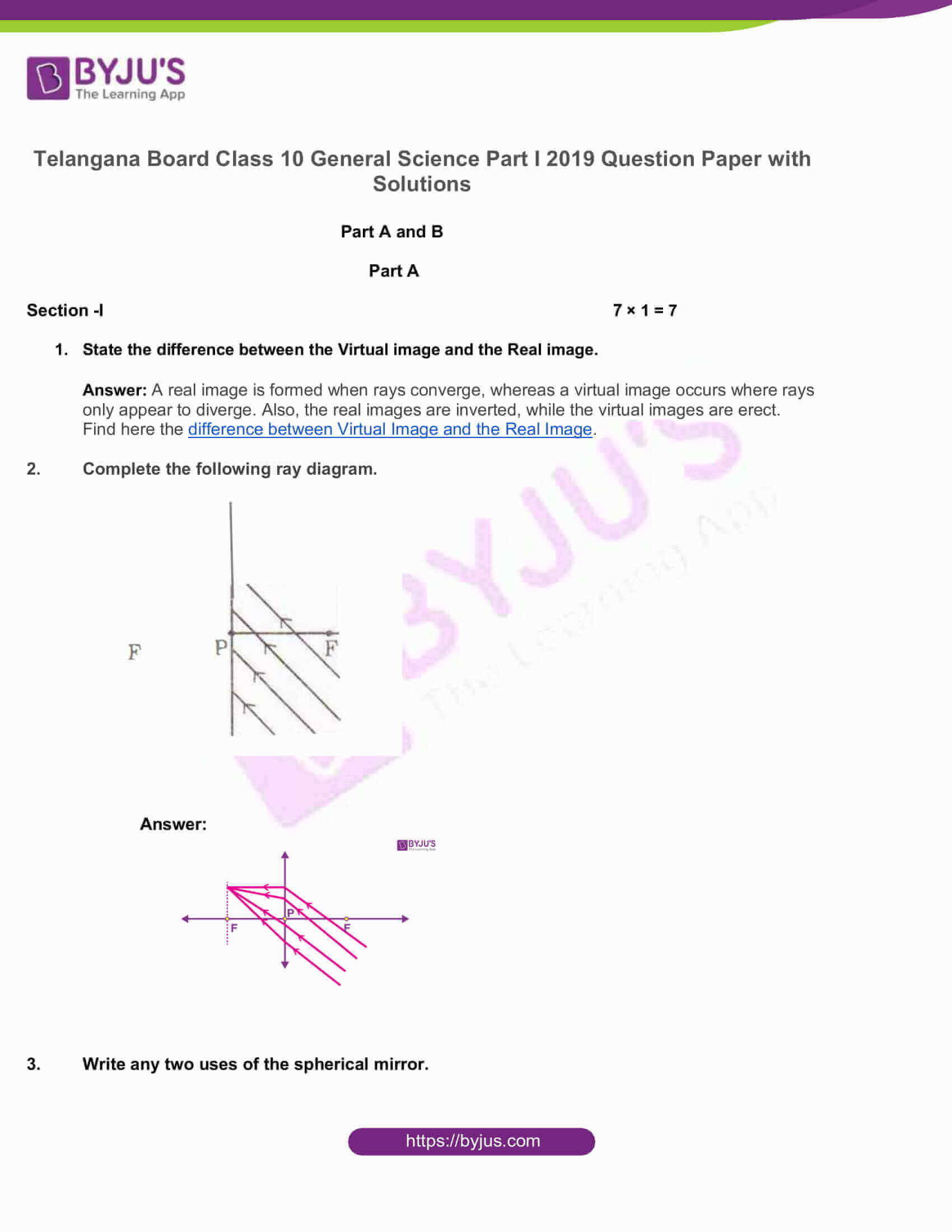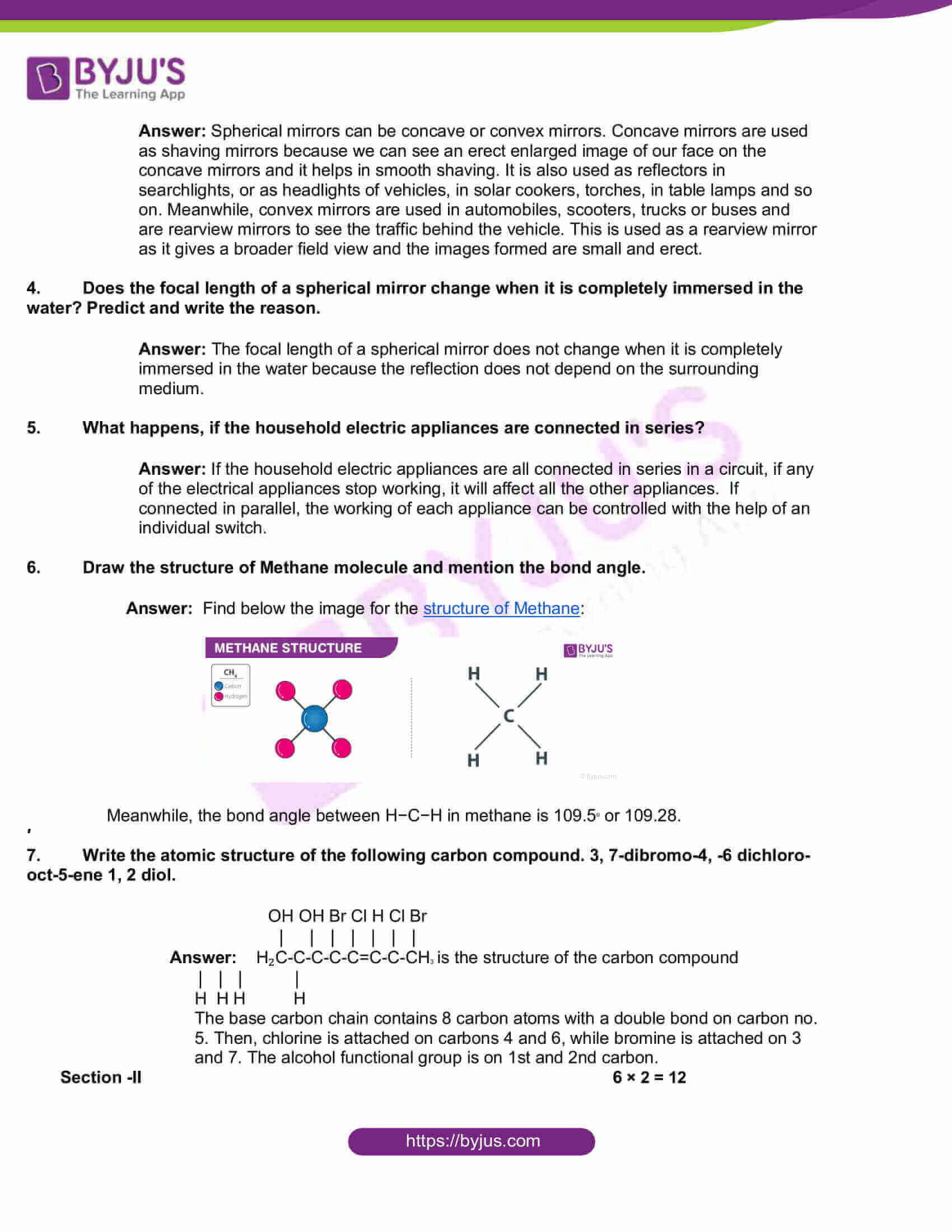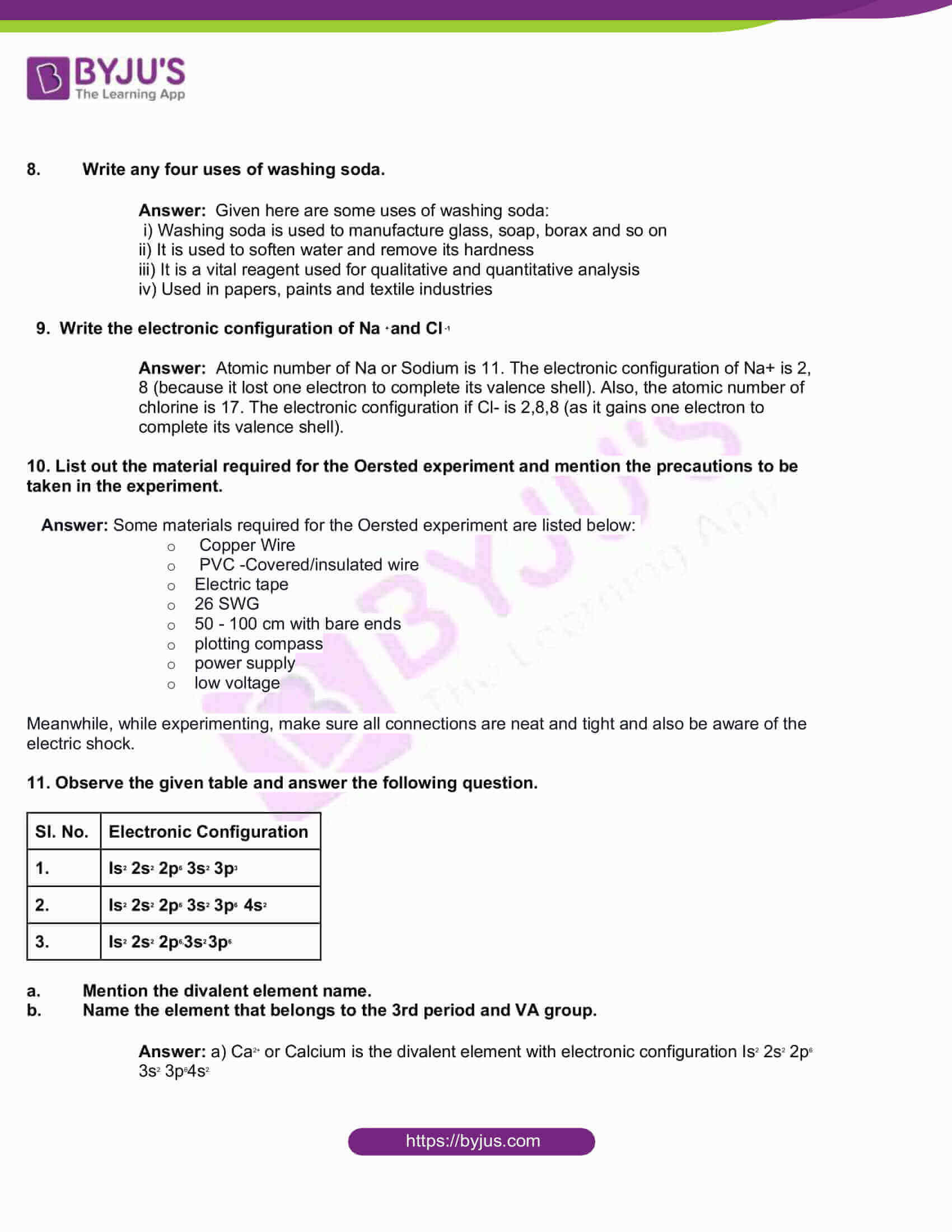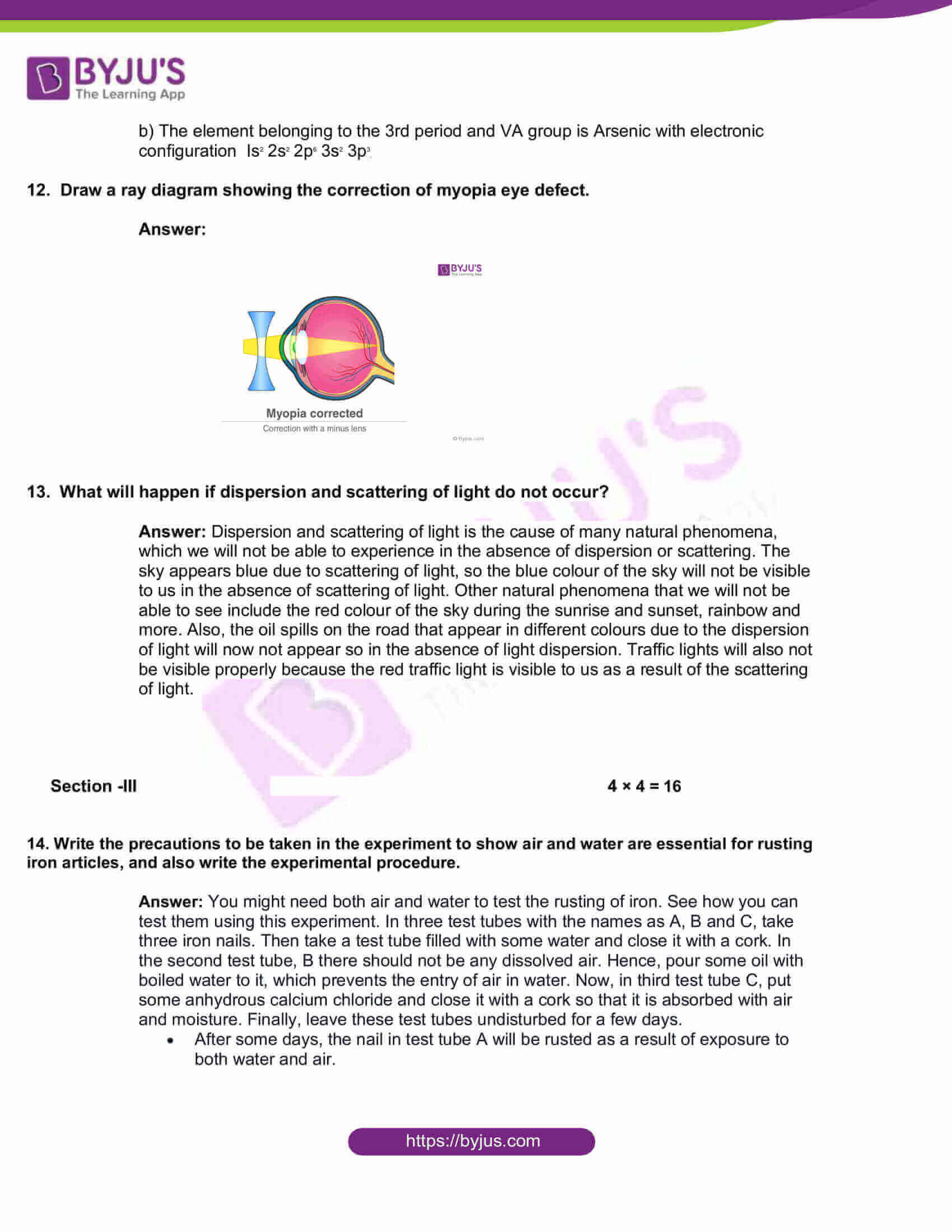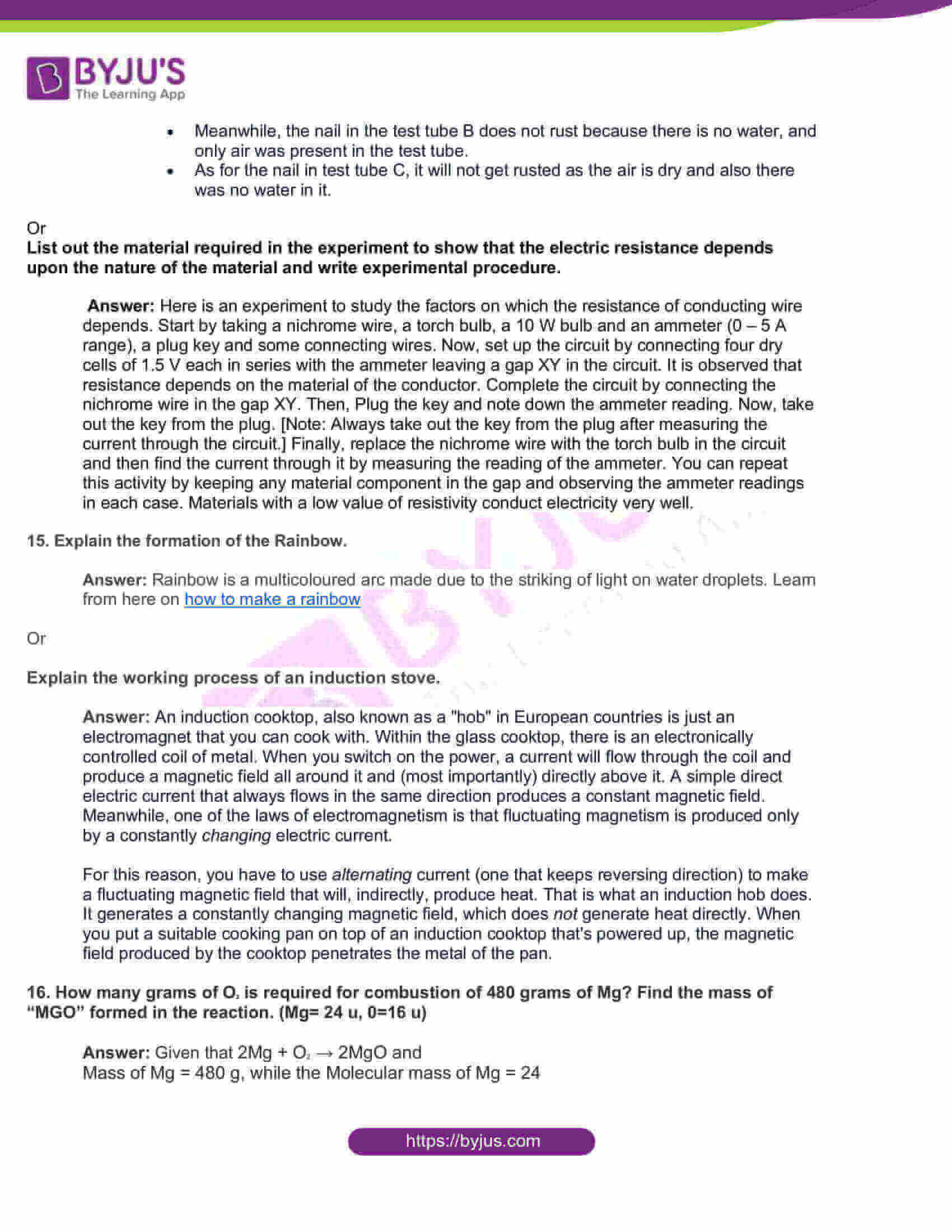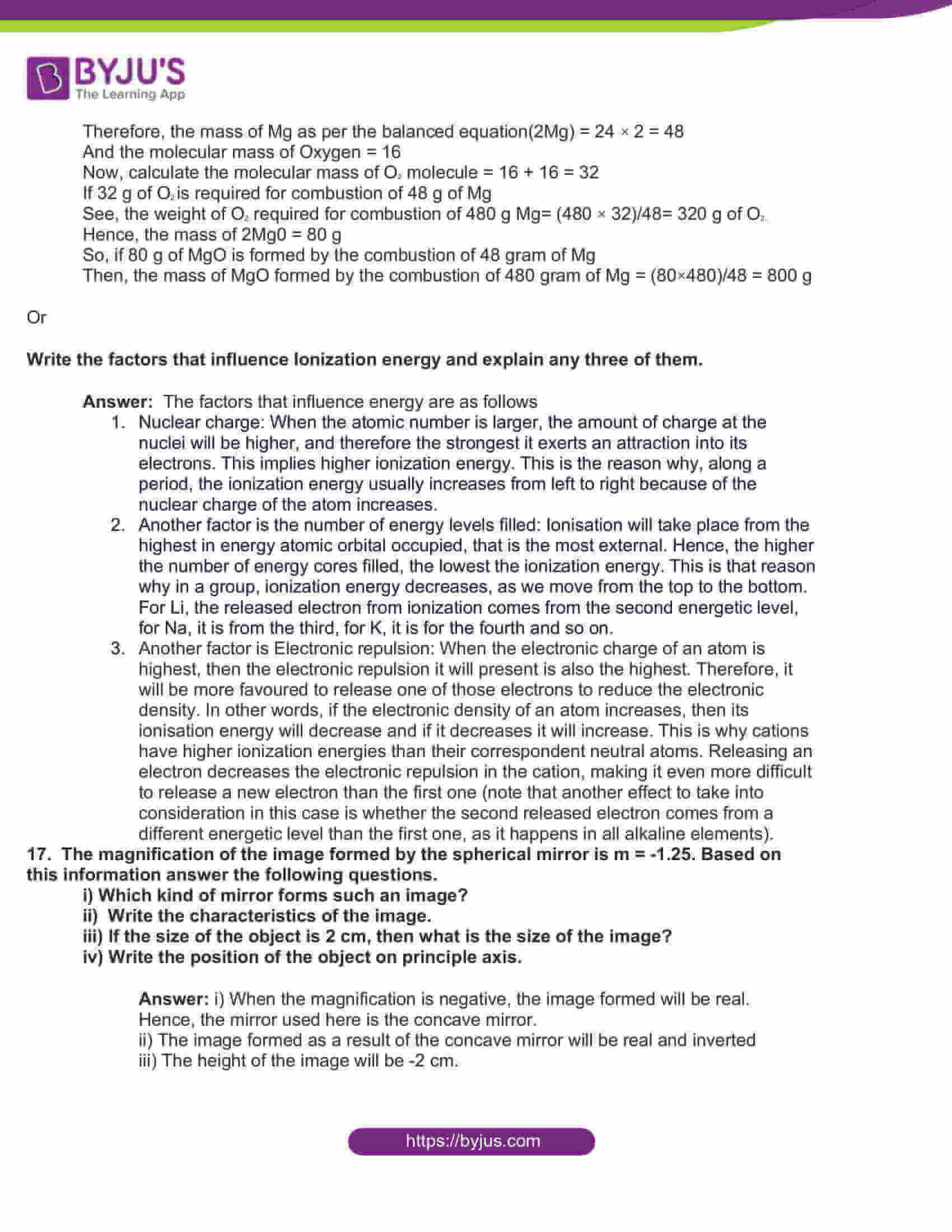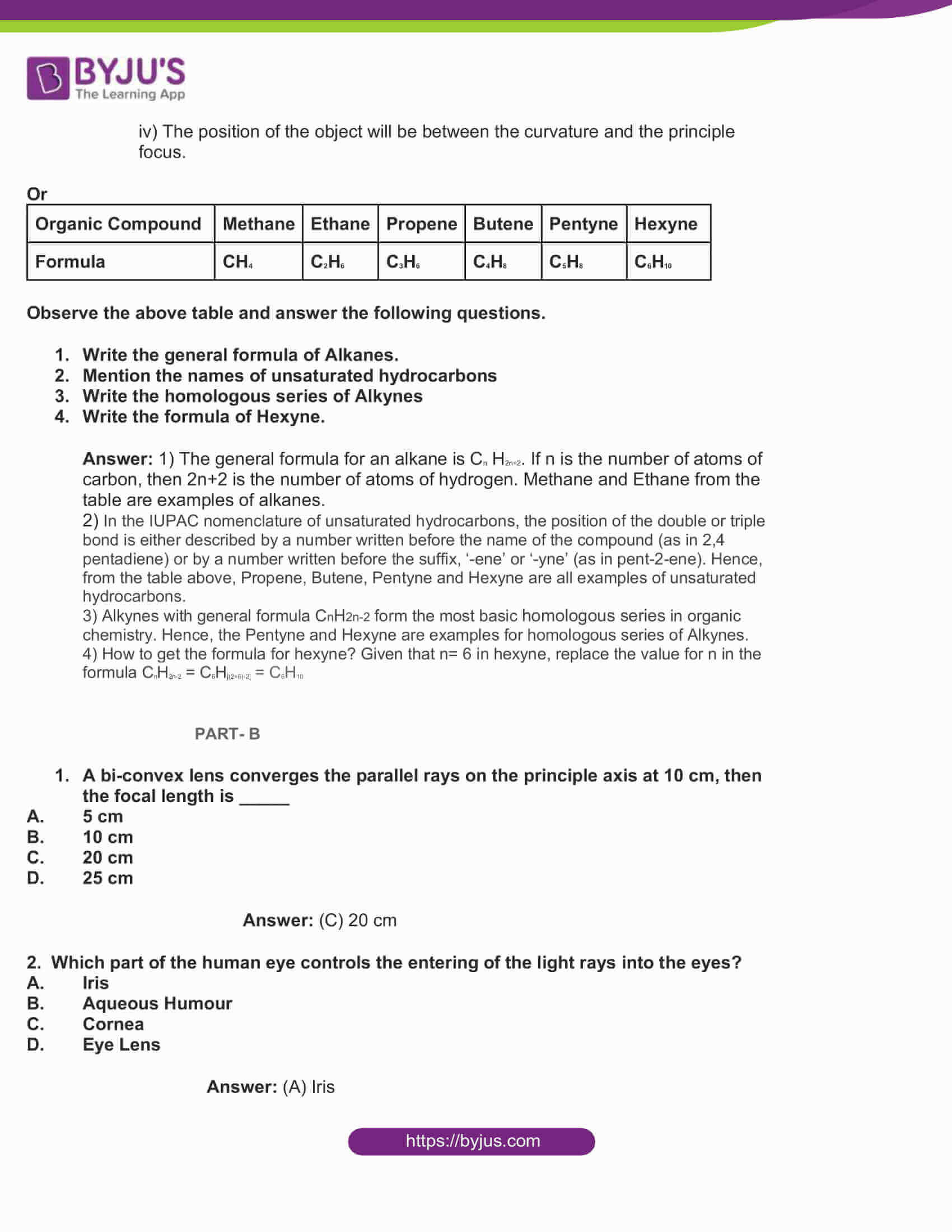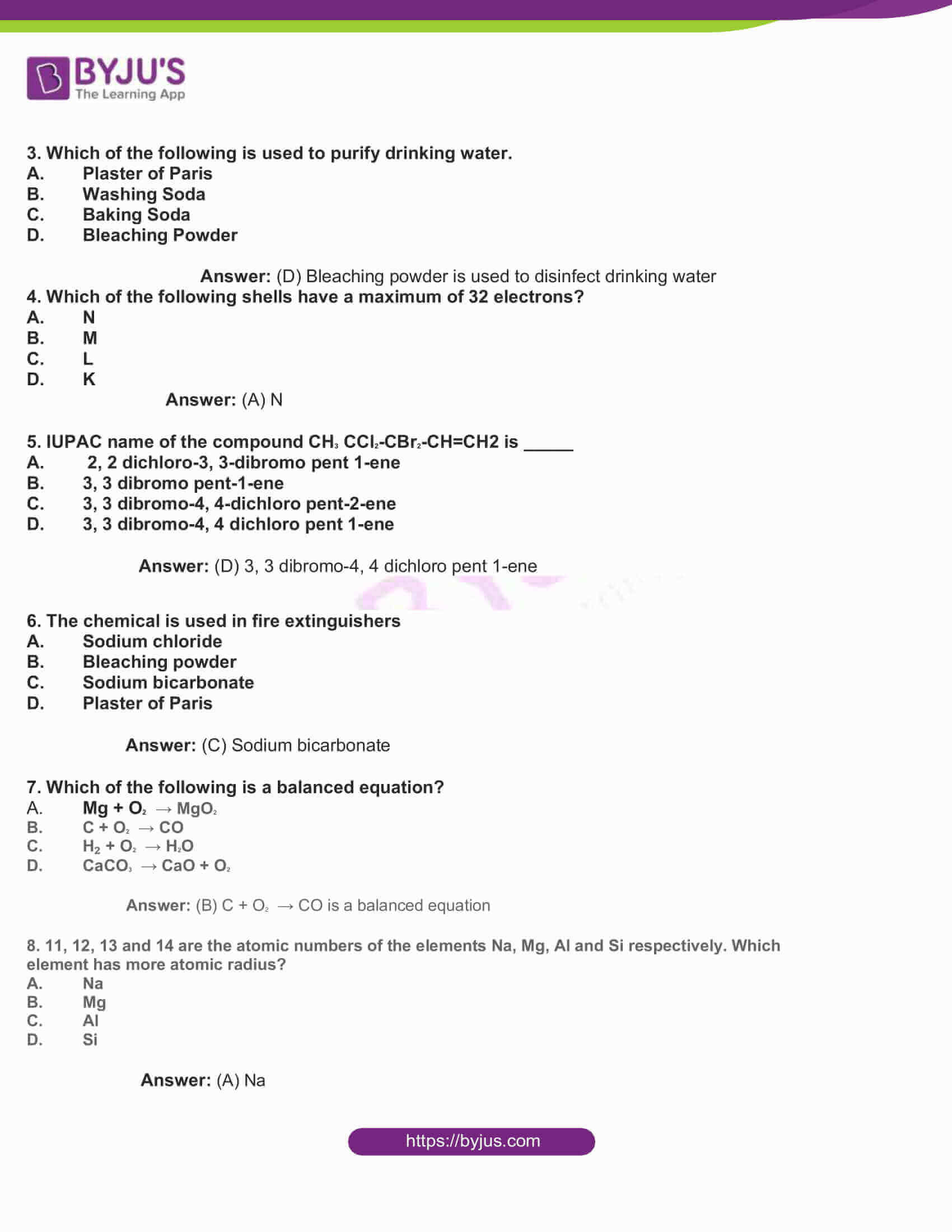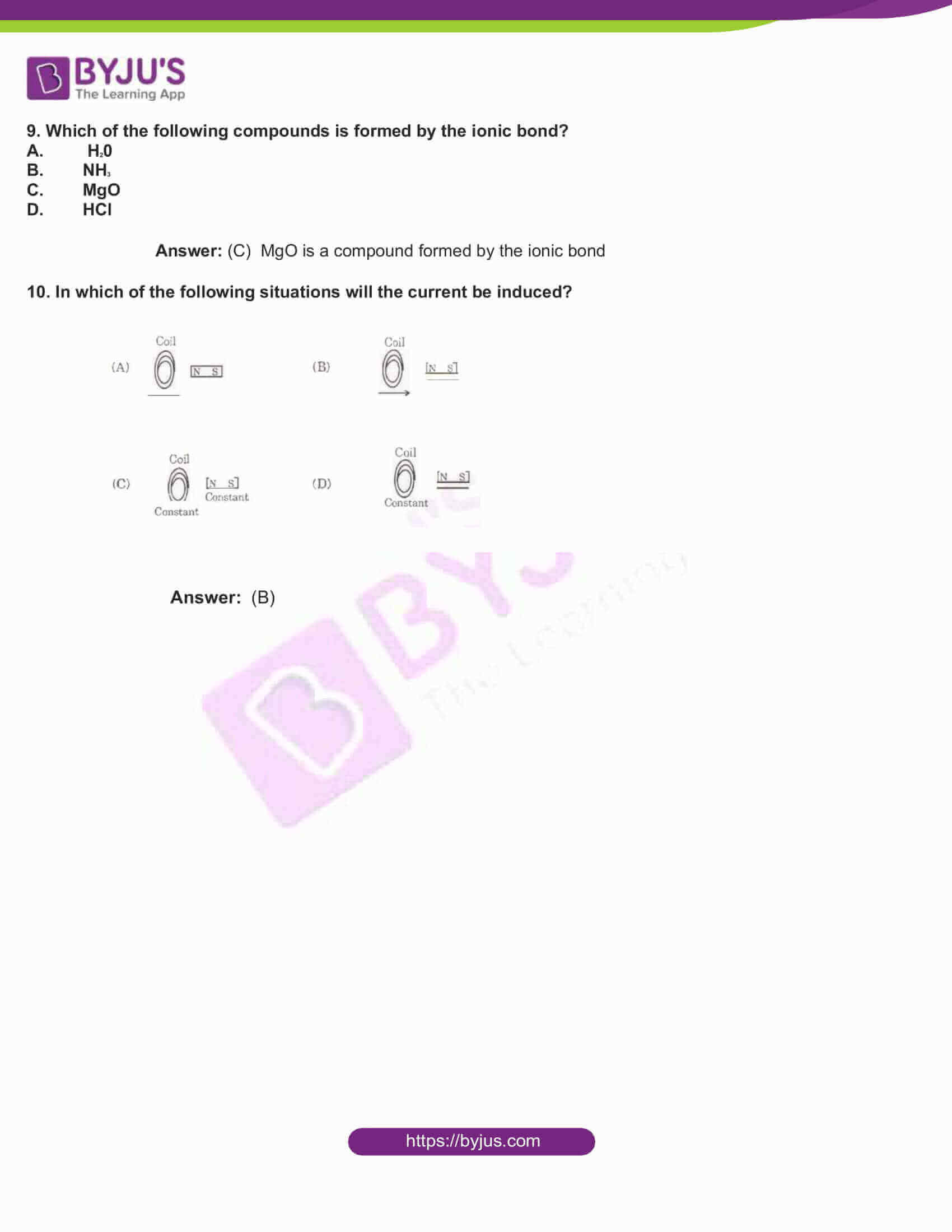Part A and B

Part A

Section -I 7 × 1 = 7

1. State the difference between the Virtual image and the Real image.

Answer: A real image is formed when rays converge, whereas a virtual image occurs where rays only appear to diverge. Also, the real images are inverted, while the virtual images are erect. Find here the difference between Virtual Image and the Real Image.

1. Complete the following ray diagram.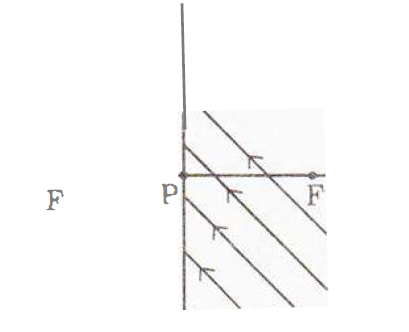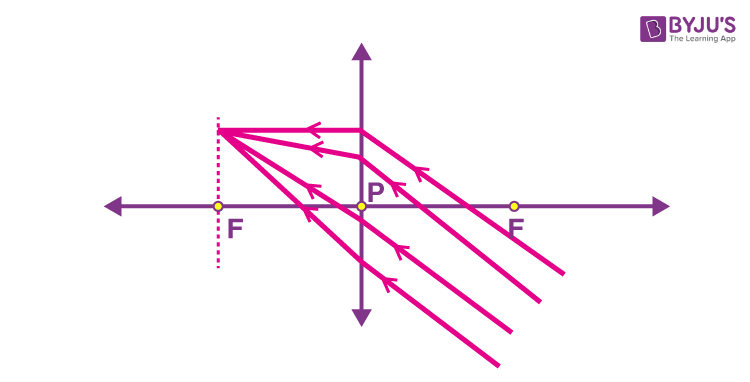1. Write any two uses of the spherical mirror.

Answer: Spherical mirrors can be concave or convex mirrors. Concave mirrors are used as shaving mirrors because we can see an erect enlarged image of our face on the concave mirrors and it helps in smooth shaving. It is also used as reflectors in searchlights, or as headlights of vehicles, in solar cookers, torches, in table lamps and so on. Meanwhile, convex mirrors are used in automobiles, scooters, trucks or buses and are rearview mirrors to see the traffic behind the vehicle. This is used as a rearview mirror as it gives a broader field view and the images formed are small and erect.

1. Does the focal length of a spherical mirror change when it is completely immersed in the water? Predict and write the reason.

Answer: The focal length of a spherical mirror does not change when it is completely immersed in the water because the reflection does not depend on the surrounding medium.

1. What happens, if the household electric appliances are connected in series?

Answer: If the household electric appliances are all connected in series in a circuit, if any of the electrical appliances stop working, it will affect all the other appliances. If connected in parallel, the working of each appliance can be controlled with the help of an individual switch.

1. Draw the structure of Methane molecule and mention the bond angle.

Answer: Find below the image for the structure of Methane: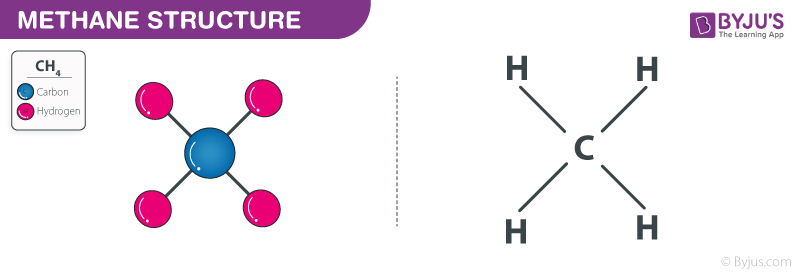Meanwhile, the bond angle between H−C−H in methane is 109.5o or 109.28.

## ′

1. Write the atomic structure of the following carbon compound. 3, 7-dibromo-4, -6 dichloro-oct-5-ene 1, 2 diol.

OH OH Br Cl H Cl Br

| | | | | | |

Answer: H₂C-C-C-C-C=C-C-CH3 is the structure of the carbon compound

| | | |

H H H H

The base carbon chain contains 8 carbon atoms with a double bond on carbon no. 5. Then, chlorine is attached on carbons 4 and 6, while bromine is attached on 3 and 7. The alcohol functional group is on 1st and 2nd carbon.

Section -II 6 × 2 = 12

1. Write any four uses of washing soda.

Answer: Given here are some uses of washing soda:

i) Washing soda is used to manufacture glass, soap, borax and so on

ii) It is used to soften water and remove its hardness

iii) It is a vital reagent used for qualitative and quantitative analysis

iv) Used in papers, paints and textile industries

9. Write the electronic configuration of Na + and Cl -1

Answer: Atomic number of Na or Sodium is 11. The electronic configuration of Na+ is 2, 8 (because it lost one electron to complete its valence shell). Also, the atomic number of chlorine is 17. The electronic configuration if Cl- is 2,8,8 (as it gains one electron to complete its valence shell).

10. List out the material required for the Oersted experiment and mention the precautions to be taken in the experiment.

Answer: Some materials required for the Oersted experiment are listed below:

• Copper Wire
• PVC -Covered/insulated wire
• Electric tape
• 26 SWG
• 50 – 100 cm with bare ends
• plotting compass
• power supply
• low voltage

Meanwhile, while experimenting, make sure all connections are neat and tight and also be aware of the electric shock.

11. Observe the given table and answer the following question.

 SI. No. Electronic Configuration 1. Is2 2s2 2p6 3s2 3p3 2. Is2 2s2 2p6 3s2 3p6 4s2 3. Is2 2s2 2p6,3s2 3p6
1. Mention the divalent element name.
2. Name the element that belongs to the 3rd period and VA group.

Answer: a) Ca2+ or Calcium is the divalent element with electronic configuration Is2 2s2 2p6 3s2 3p64s2

b) The element belonging to the 3rd period and VA group is Arsenic with electronic configuration Is2 2s2 2p6 3s2 3p3.

12. Draw a ray diagram showing the correction of myopia eye defect.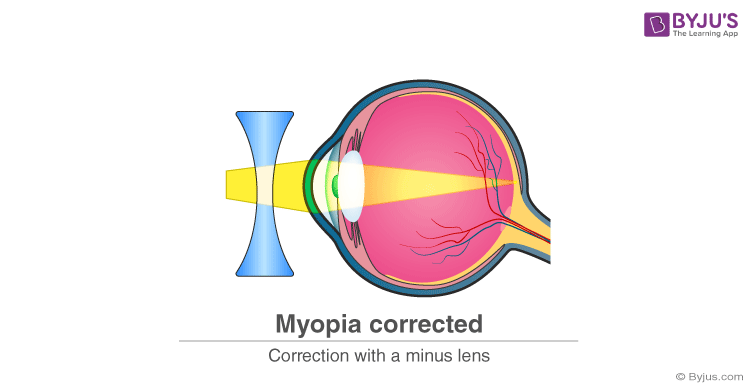13. What will happen if dispersion and scattering of light do not occur?

Answer: Dispersion and scattering of light is the cause of many natural phenomena, which we will not be able to experience in the absence of dispersion or scattering. The sky appears blue due to scattering of light, so the blue colour of the sky will not be visible to us in the absence of scattering of light. Other natural phenomena that we will not be able to see include the red colour of the sky during the sunrise and sunset, rainbow and more. Also, the oil spills on the road that appear in different colours due to the dispersion of light will now not appear so in the absence of light dispersion. Traffic lights will also not be visible properly because the red traffic light is visible to us as a result of the scattering of light.

Section -III 4 × 4 = 16

14. Write the precautions to be taken in the experiment to show air and water are essential for rusting iron articles, and also write the experimental procedure.

Answer: You might need both air and water to test the rusting of iron. See how you can test them using this experiment. In three test tubes with the names as A, B and C, take three iron nails. Then take a test tube filled with some water and close it with a cork. In the second test tube, B there should not be any dissolved air. Hence, pour some oil with boiled water to it, which prevents the entry of air in water. Now, in third test tube C, put some anhydrous calcium chloride and close it with a cork so that it is absorbed with air and moisture. Finally, leave these test tubes undisturbed for a few days.

• After some days, the nail in test tube A will be rusted as a result of exposure to both water and air.
• Meanwhile, the nail in the test tube B does not rust because there is no water, and only air was present in the test tube.
• As for the nail in test tube C, it will not get rusted as the air is dry and also there was no water in it.

Or

List out the material required in the experiment to show that the electric resistance depends upon the nature of the material and write experimental procedure.

Answer: Here is an experiment to study the factors on which the resistance of conducting wire depends. Start by taking a nichrome wire, a torch bulb, a 10 W bulb and an ammeter (0 – 5 A range), a plug key and some connecting wires. Now, set up the circuit by connecting four dry cells of 1.5 V each in series with the ammeter leaving a gap XY in the circuit. It is observed that resistance depends on the material of the conductor. Complete the circuit by connecting the nichrome wire in the gap XY. Then, Plug the key and note down the ammeter reading. Now, take out the key from the plug. [Note: Always take out the key from the plug after measuring the current through the circuit.] Finally, replace the nichrome wire with the torch bulb in the circuit and then find the current through it by measuring the reading of the ammeter. You can repeat this activity by keeping any material component in the gap and observing the ammeter readings in each case. Materials with a low value of resistivity conduct electricity very well.

15. Explain the formation of the Rainbow.

Answer: Rainbow is a multicoloured arc made due to the striking of light on water droplets. Learn from here on how to make a rainbow

Or

Explain the working process of an induction stove.

Answer: An induction cooktop, also known as a “hob” in European countries is just an electromagnet that you can cook with. Within the glass cooktop, there is an electronically controlled coil of metal. When you switch on the power, a current will flow through the coil and produce a magnetic field all around it and (most importantly) directly above it. A simple direct electric current that always flows in the same direction produces a constant magnetic field. Meanwhile, one of the laws of electromagnetism is that fluctuating magnetism is produced only by a constantly changing electric current.

For this reason, you have to use alternating current (one that keeps reversing direction) to make a fluctuating magnetic field that will, indirectly, produce heat. That is what an induction hob does. It generates a constantly changing magnetic field, which does not generate heat directly. When you put a suitable cooking pan on top of an induction cooktop that’s powered up, the magnetic field produced by the cooktop penetrates the metal of the pan.

16. How many grams of O2 is required for combustion of 480 grams of Mg? Find the mass of “MGO” formed in the reaction. (Mg= 24 u, 0=16 u)

Answer: Given that 2Mg + O2 → 2MgO and

Mass of Mg = 480 g, while the Molecular mass of Mg = 24

Therefore, the mass of Mg as per the balanced equation(2Mg) = 24 × 2 = 48

And the molecular mass of Oxygen = 16

Now, calculate the molecular mass of O2 molecule = 16 + 16 = 32

If 32 g of O2 is required for combustion of 48 g of Mg

See, the weight of O2 required for combustion of 480 g Mg= (480 × 32)/48= 320 g of O2 . Hence, the mass of 2Mg0 = 80 g

So, if 80 g of MgO is formed by the combustion of 48 gram of Mg

Then, the mass of MgO formed by the combustion of 480 gram of Mg = (80×480)/48 = 800 g

Or

Write the factors that influence Ionization energy and explain any three of them.

Answer: The factors that influence energy are as follows

1. Nuclear charge: When the atomic number is larger, the amount of charge at the nuclei will be higher, and therefore the strongest it exerts an attraction into its electrons. This implies higher ionization energy. This is the reason why, along a period, the ionization energy usually increases from left to right because of the nuclear charge of the atom increases.
2. Another factor is the number of energy levels filled: Ionisation will take place from the highest in energy atomic orbital occupied, that is the most external. Hence, the higher the number of energy cores filled, the lowest the ionization energy. This is that reason why in a group, ionization energy decreases, as we move from the top to the bottom. For Li, the released electron from ionization comes from the second energetic level, for Na, it is from the third, for K, it is for the fourth and so on.
3. Another factor is Electronic repulsion: When the electronic charge of an atom is highest, then the electronic repulsion it will present is also the highest. Therefore, it will be more favoured to release one of those electrons to reduce the electronic density. In other words, if the electronic density of an atom increases, then its ionisation energy will decrease and if it decreases it will increase. This is why cations have higher ionization energies than their correspondent neutral atoms. Releasing an electron decreases the electronic repulsion in the cation, making it even more difficult to release a new electron than the first one (note that another effect to take into consideration in this case is whether the second released electron comes from a different energetic level than the first one, as it happens in all alkaline elements).

17. The magnification of the image formed by the spherical mirror is m = -1.25. Based on this information answer the following questions.

i) Which kind of mirror forms such an image?

ii) Write the characteristics of the image.

iii) If the size of the object is 2 cm, then what is the size of the image?

iv) Write the position of the object on principle axis.

Answer: i) When the magnification is negative, the image formed will be real. Hence, the mirror used here is the concave mirror.

ii) The image formed as a result of the concave mirror will be real and inverted

iii) The height of the image will be -2 cm.

iv) The position of the object will be between the curvature and the principle focus.

Or

 Organic Compound Methane Ethane Propene Butene Pentyne Hexyne Formula CH4 C2H6 C3H6 C4H8 C5H8 C6H10

Observe the above table and answer the following questions.

1. Write the general formula of Alkanes.
2. Mention the names of unsaturated hydrocarbons
3. Write the homologous series of Alkynes
4. Write the formula of Hexyne.

Answer: 1) The general formula for an alkane is Cn H2n+2. If n is the number of atoms of carbon, then 2n+2 is the number of atoms of hydrogen. Methane and Ethane from the table are examples of alkanes.

2) In the IUPAC nomenclature of unsaturated hydrocarbons, the position of the double or triple bond is either described by a number written before the name of the compound (as in 2,4 pentadiene) or by a number written before the suffix, ‘-ene’ or ‘-yne’ (as in pent-2-ene). Hence, from the table above, Propene, Butene, Pentyne and Hexyne are all examples of unsaturated hydrocarbons.

3) Alkynes with general formula CnH2n-2 form the most basic homologous series in organic chemistry. Hence, the Pentyne and Hexyne are examples for homologous series of Alkynes.

4) How to get the formula for hexyne? Given that n= 6 in hexyne, replace the value for n in the formula CnH2n-2 = C6H[(2×6)-2] = C6H10

PART- B

1. A bi-convex lens converges the parallel rays on the principle axis at 10 cm, then the focal length is _____
1. 5 cm
2. 10 cm
3. 20 cm
4. 25 cm

2. Which part of the human eye controls the entering of the light rays into the eyes?

1. Iris
2. Aqueous Humour
3. Cornea
4. Eye Lens

3. Which of the following is used to purify drinking water.

1. Plaster of Paris
2. Washing Soda
3. Baking Soda
4. Bleaching Powder

Answer: (D) Bleaching powder is used to disinfect drinking water

4. Which of the following shells have a maximum of 32 electrons?

1. N
2. M
3. L
4. K

5. IUPAC name of the compound CH3 CCl2-CBr2-CH=CH2 is _____

1. 2, 2 dichloro-3, 3-dibromo pent 1-ene
2. 3, 3 dibromo pent-1-ene
3. 3, 3 dibromo-4, 4-dichloro pent-2-ene
4. 3, 3 dibromo-4, 4 dichloro pent 1-ene

Answer: (D) 3, 3 dibromo-4, 4 dichloro pent 1-ene

6. The chemical is used in fire extinguishers

1. Sodium chloride
2. Bleaching powder
3. Sodium bicarbonate
4. Plaster of Paris

7. Which of the following is a balanced equation?

1. Mg + O2 → MgO2
2. C + O2 → CO
3. H₂ + O2 → H2O
4. CaCO3 → CaO + O2

Answer: (B) C + O2 → CO is a balanced equation

8. 11, 12, 13 and 14 are the atomic numbers of the elements Na, Mg, Al and Si respectively. Which element has more atomic radius?

1. Na
2. Mg
3. Al
4. Si

9. Which of the following compounds is formed by the ionic bond?

1. H20
2. NH3
3. MgO
4. HCl

Answer: (C) MgO is a compound formed by the ionic bond

10. In which of the following situations will the current be induced?